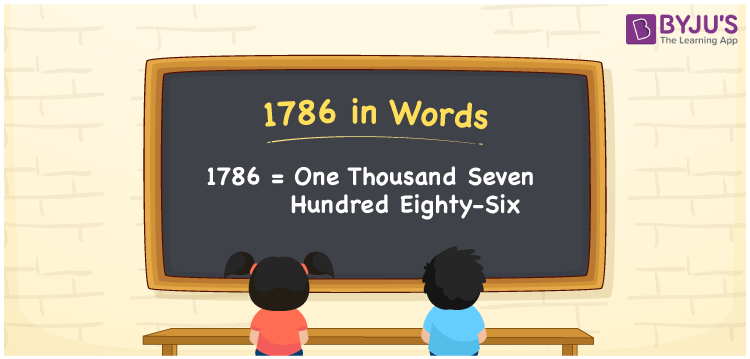# 1786 in Words

1786 in words is written as One Thousand Seven Hundred Eighty-Six. For example, the cost of a jacket is Rs. 1786, then you can say, “The cost of a jacket is Rupees One Thousand Seven Hundred Eighty-Six”. 1786 is a cardinal number since it depicts a specific quantity. We can easily convert any number into words with the help of a place value system. The technique of converting the number 1786 into word form using a place value table is provided with a complete explanation in this article.

 1786 in Words One Thousand Seven Hundred Eighty-Six One Thousand Seven Hundred Eighty-Six in numerical form 1786

## 1786 in English Words

Generally, in Mathematics, we write numbers in words using the letters of the English alphabet. Therefore, we can express the number 1786 in English as One Thousand Seven Hundred Eighty-Six.## How to Write 1786 in Words?

We can convert the number 1786 into words using a place value table with ease. The following table represents the place value chart for 1786.

 Thousands Hundreds Tens Ones 1 7 8 6

Hence, we can write the expanded form as:

1 x Thousand + 7 x Hundred + 8 x Ten + 6 x One

= 1 x 1000 + 7 x 100 + 8 x 10 + 6 x 1

= 1000 + 700 + 80 + 6

= 1786

= One Thousand Seven Hundred Eighty-Six

Therefore, 1786 in words is written as One Thousand Seven Hundred Eighty-Six

Interesting way of writing 1786 in words

1 = One

17 = Seventeen

178 = One Hundred and Seventy-Eight

1786 = One Thousand Seven Hundred Eighty-Six

Thus, the word form of the number 1786 is One Thousand Seven Hundred Eighty-Six

1786 is a natural number that precedes 1787 and succeeds 1785

• 1786 in words – One Thousand Seven Hundred Eighty-Six
• Is 1786 an odd number? – No
• Is 1786 an even number? – Yes
• Is 1786 a perfect square number? – No
• Is 1786 a perfect cube number? – No
• Is 1786 a prime number? – No
• Is 1786 a composite number? – Yes

## Frequently Asked Questions on 1786 in Words

Q1

### How to write 1786 in words?

We can write 1786 in words as One Thousand Seven Hundred Eighty-Six.
Q2

### Simplify 1000 + 786, and express in words.

Simplifying 1000 + 786, we get 1786. Therefore, the number 1786 in words is One Thousand Seven Hundred Eighty-Six.
Q3

### Is 1786 a prime number?

No, the number 1786 is not a prime number.